# Effects of system topologies’ attributes on average consensus algorithm - part II.

Autor: M. Kenyeres, T. Danhel, J. Kenyeres, V. Škorpil <kenyeres(at)phd.feec.vutbr.cz>, Pracoviště: Vysoké učení technické v Brně, Téma: Aplikace, sítě a služby, Vydáno dne: 24. 06. 2015

In this publication, we focused our attention on examining the effects of the topology on the average consensus algorithm. We examined the number of the necessary iterations for a system to achieve the consensus while altering several parameters: the speed of the algoritm convergence (ε), dispersion and shift of the initial values. In our analysis, we considered networkspokracovanie with the tree, ring, star and mesh topology. At the end, we compared the obtained results.

Keywords: distributed computing; average consensus; ring/star/tree/mesh topologies

### Introduction

This paper is a continuance of the publication titled as Effects of system topologies’ attributes on average consensus algorithm I. In this part, we examined how the dispersion of the initial values and a shift of the initial values affected the number of the iterations necessary for a network to achieve the consensus il. The theorethical basis is descibed in .

### Experiments

Also in this part, we examined how the topology of a distributed system affected the features of average consensus algorithm. We chose four topologies with the same size of 32 elements. The networks are of a tree, ring, star and mesh topology. We altered two parameters: the dispersion of the initial values and the shift of the initial values and examined how these parameters affected il.

### The effect of the dispersion

In the third experiment, we examined how the dispersion of data would affect il. The dispersion was achieved applying the formula (1). The results are shown in the followings graphs.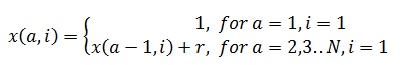(1)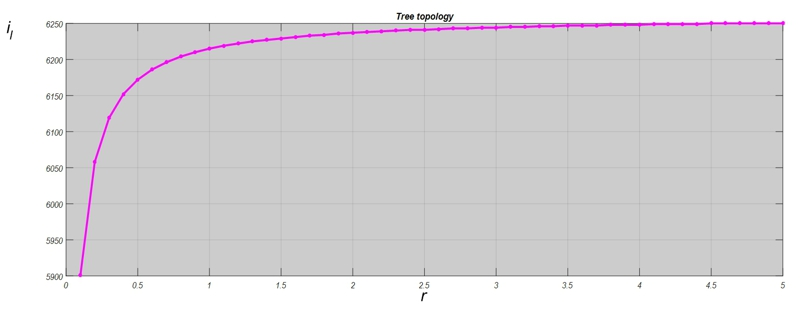Fig. 1 Impact of changes of r - tree topology

In the tree topology, we can see that the function grows fast for small r. Then for increasing r, the function's growth is minimal.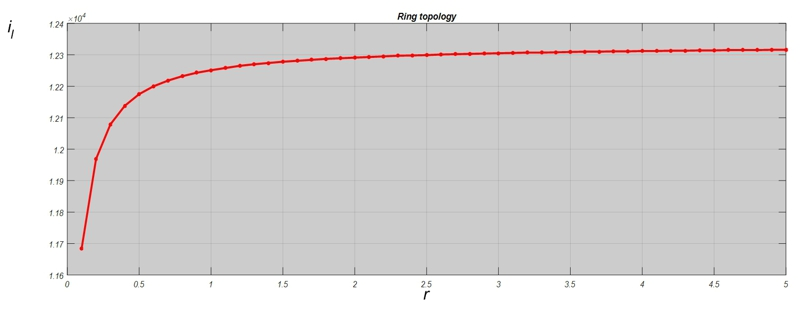Fig. 2 Impact of changes of r - ring topology

In the ring topology, average consensus behaves like in the previous case. The growth is significantly minimized when r reaches the higher values.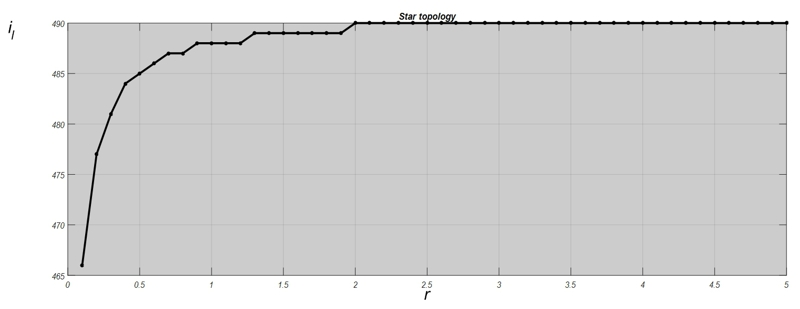Fig. 3 Impact of changes of r - star topology

When we executed this experiment in the network of the star topology, we can see the result similar to the previous cases. However, there are intervals during which the function is constant and so, il does not change continually. The function is not as smooth as it was in the previous cases.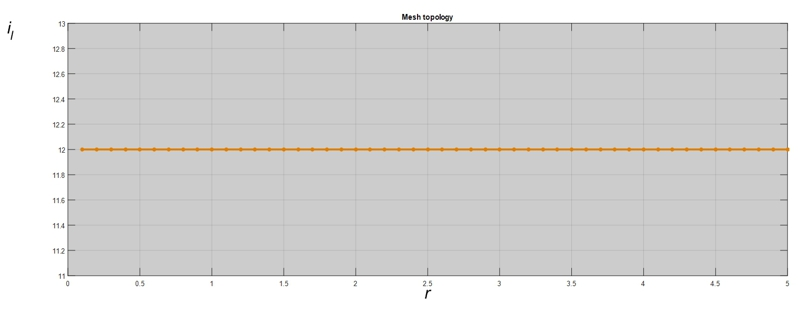Fig. 4 Impact of changes of r - mesh topology

In the mesh topology, the parameter r does not have any effect. The function is constant for all the values of r.Fig. 5 The relative comparision of the results from the second experiment

### Impact of the initial shift

In the last experiment, we examined the effect of the parameter p (defined according to (2)) which increases the initial values' size without causing the data dispersion. The results have been depicted in the following graphs.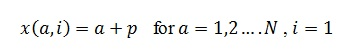(2)

For each topology, the functions is decreasing as p is increasing.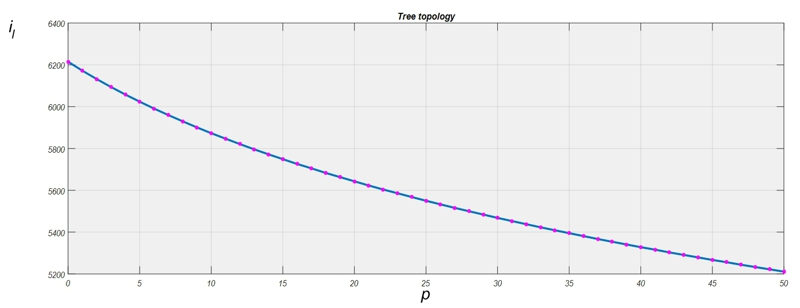Fig. 6 Impact of changes of p - tree topology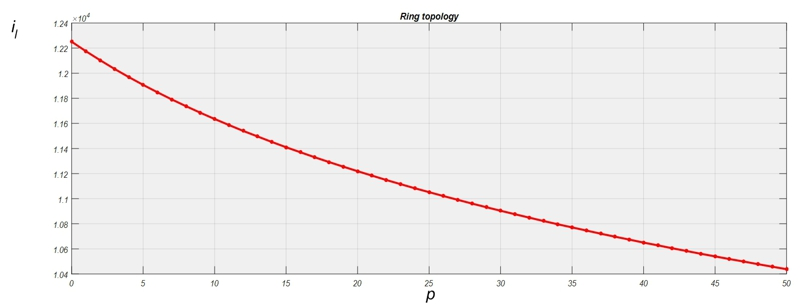Fig. 7 Impact of changes of p - ring topologyFig. 8 Impact of changes of p - star topology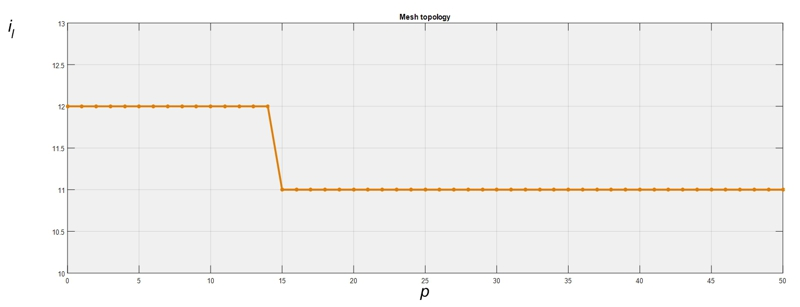Fig. 9 Impact of changes of p - mesh topology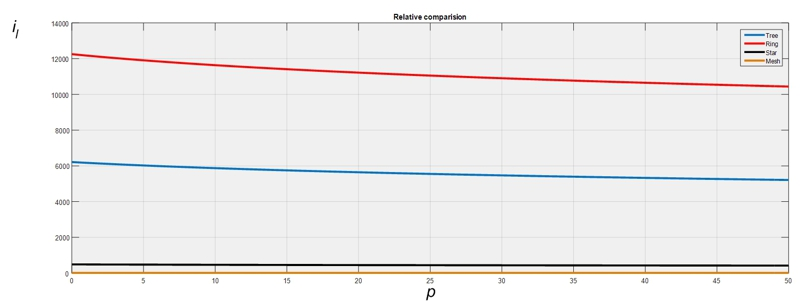Fig. 10 The relative comparision of the results from the third experiment

### Contribution

In this paper, we examined the effect dispersion of the initial values and their shift on the features of the average consensus in the networks with the tree, ring, star and mesh topology. From the results, we can see that the network behviour is very similar. The only exception is the mesh topology, which is very specific in the terms of the connectivity.

### Acknowledgment

Research described in this paper was financed by the National Sustainability Program under grant LO1401. For the research, infrastructure of the SIX Center was used.

### Literature

 Kenyeres, M., Danhel, T., Kenyeres, J., Škorpil, V.: Effects of system topologies’ attributes on average consensus algorithm I. Access server on-line. Praha 2015.